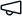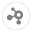Give us feedback# Url Shorten

Stable version 1.0.2 (Compatible with OutSystems 11)
0.0
(0 ratings)# Url Shorten

Details
This will help you URL shorten service where you can write a long URL into an input field and the service shortens the URL to "http://www.example.org/abcdef".

I have a database table with three columns:

1. id, integer, auto-increment
2. long, string, the long URL the user entered
3. short, string, the shortened URL (or just the six characters)

Demo Url :Theoretical background

You need a Bijective Function f. This is necessary so that you can find a inverse function g('abc') = 123 for your f(123) = 'abc' function. This means:

There must be no x1, x2 (with x1 ? x2) that will make f(x1) = f(x2),

and for every y you must be able to find an x so that f(x) = y.

How to convert the ID to a shortened URL

Think of an alphabet we want to use. In your case, that's [a-zA-Z0-9]. It contains 62 letters.

Take an auto-generated, unique numerical key (the auto-incremented id of a MySQL table for example).

For this example, I will use 12510 (125 with a base of 10).

Now you have to convert 12510 to X62 (base 62).

12510 = 2×621 + 1×620 = [2,1]

This requires the use of integer division and modulo. A pseudo-code example:

digits = []

while num > 0

remainder = modulo(num, 62)

digits.push(remainder)

num = divide(num, 62)

digits = digits.reverse

Now map the indices 2 and 1 to your alphabet. This is how your mapping (with an array for example) could look like:

0  ? a

1  ? b

...

25 ? z

...

52 ? 0

61 ? 9

With 2 ? c and 1 ? b, you will receive cb62 as the shortened URL.

http://shor.ty/cb

How to resolve a shortened URL to the initial ID

The reverse is even easier. You just do a reverse lookup in your alphabet.

e9a62 will be resolved to "4th, 61st, and 0th letter in the alphabet".

e9a62 = [4,61,0] = 4×622 + 61×621 + 0×620 = 1915810

Now find your database-record with WHERE id = 19158 and do the redirect.

Example implementations (provided by commenters)

C++

Python

Ruby

C#

CoffeeScript

Perl

Release notes (1.0.2)

We did the below changes in the components

1. Changes in report Screen
2. Create an API module for configuring the domain.
3. Convert Asset type to service